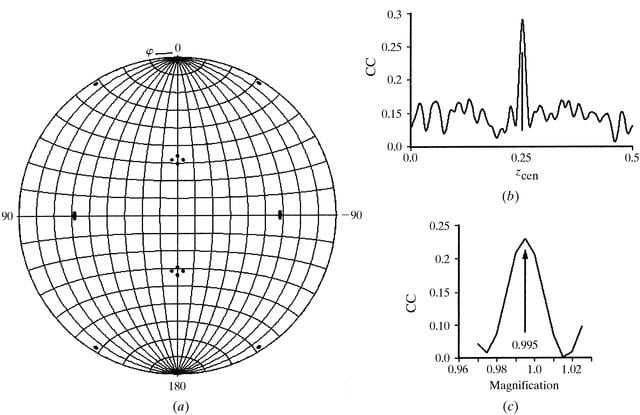disable zoom     view article Figure 6 Molecular replacement solution for the core particle of BTV-1 SA. (a) The κ = 72° section of the self-rotation function. Patterson vectors between 600 and 200 Å in length were used in the integration, and the 125000 highest peaks were used in the search. The sharp peaks define the orientations of the fivefold axes extremely accurately. (b) The translation search of the crude model along the z-axis. Data between 60 and 30 Å were used in the calculation and the search was from 0 to 0.5 in steps of 2 Å along the z-axis. (c) The amplification search for optimization of the cryo-EM data to the crystallographic data between 25 and 20 Å. A grid search was used where model coordinates were scaled by a factor of 0.96 to 1.03 in steps of 0.005, showing a clear peak with a scale factor of 0.995. CC is the linear correlation coefficient calculated between observed and calculated structure factors, zcen is the fractional z coordinate for the particle centre and magnification is the magnification of the cryo-EM data.JOURNAL OFSYNCHROTRONRADIATION
ISSN: 1600-5775
Volume 6| Part 4| July 1999| Pages 865-874